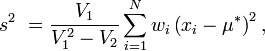# Weighted variance and weighted coefficient of variation

Often we want to compare the variability of a variable in different contexts – say, the variability of unemployment in different countries over time, or the variability of height in two populations, etc. The most often used measures of variability are the variance and the standard deviation (which is just the square root of the variance). However, for some types of data, these measures are not entirely appropriate. For example, when data is generated by a Poisson process (e.g. when you have counts of rare events) the mean equals the variance by definition. Clearly, comparing the variability of two Poisson distributions using the variance or the standard deviation would not work if the means of these populations differ. A common and easy fix is to use the coefficient of variation instead, which is simply the standard deviation divided by the mean. So far, so good.

Things get tricky however when we want to calculate the weighted coefficient of variation. The weighted mean is just the mean but some data points contribute more than others. For example the mean of 0.4 and 0.8 is 0.6. If we assign the weights 0.9 to the first observation [0.4] and 0.1 to the second [0.8], the weighted mean is (0.9*0.4+0.1*0.8)/1, which equals to 0.44. You would guess that we can compute the weighted variance by analogy,  and you would be wrong.

For example, the sample variance of {0.4,0.8} is given by [Wikipedia]:or in our example ((0.4-0.6)^2+(0.8-0.6)^2) / (2-1) which equals to 0.02. But, the weighted sample variance cannot be computed by simply adding the weights to the above formula (0.9*(0.4-0.6)^2+0.1*(0.8-0.6)^2) / (2-1). The formula for the weighted variance is different [Wikipedia]:where V1 is the sum of the weights and V2 is the sum of squared weights:.
The next steps are straightforward: the weighted standard deviation is the square root of the above, and the weighted coefficient of variation is the weighted standard deviation divided by the weighted mean.

Although there is nothing new here, I thought it’s a good idea to put it together because it appears to be causing some confusion.  For example, in the latest issue of European Union Politics you can find the article ‘Measuring common standards  and equal responsibility-sharing in EU asylum outcome data’  by a team of scientists from LSE. On page 74, you can read that:

The weighted variance [of the set p={0.38, 0.42} with weights W={0.50,0.50}] equals 0.5(0.38-.0.40)^2+0.5(0.42-0.40)^2 =0.0004.

As explained above, this is not generally correct unless the biased (population) rather than the unbiased (sample)  weighted variance is meant. When calculated properly, the weighted variance turns out to be 0.0008. Here you can find the function Gavin Simpson has provided  for calculating the weighted variance in R and try for yourself.

P.S. To be clear, the weighted variance issue is not central to the argument of the article cited above but is significant as the authors discuss at length the methodology for estimating variability in data and introduce the so-called Coffey-Feingold-Broomberg measure of variability which the authors  deem more appropriate for proportions.

P.P.S On the internet, there is yet more confusion: for example, this document (which pops high in the Google results) has yet a different formula, shown in a slightly different form here  as  well.

Disclaimer. I have a forthcoming paper on the same topic (asylum policy) as the EUP article mentioned above.

## 5 thoughts on “Weighted variance and weighted coefficient of variation”

1. Pato says:

Hi, I’ve been taking a look at this calculation also, for a work I’m doing. Have you find a reference for the weighted variance (s^2) formula? any book or paper? thanks!

2. Nice post. Do you know any R package that has a build in function for the weighted variance?

3. AA says:

Hi Dimiter,

It’s interesting how the two pages you’ve linked in PS and PPS were ones I’ve come across in the same search, but I’d like to add another one: http://stackoverflow.com/questions/2413522/weighted-standard-deviation-in-numpy

This one is related to a python question seeking the same answer.

I’m curious if the average taken in this function to calculated the variance should not be the weighted average but simply the average of the values? The results matches up to your article otherwise when the average used to calculate the variance isn’t weighted.